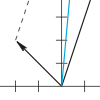# The Geometry of Linear Equations

« Previous | Next »

## Session OverviewA major application of linear algebra is to solving systems of linear equations. This lecture presents three ways of thinking about these systems. The “row method” focuses on the individual equations, the “column method” focuses on combining the columns, and the “matrix method” is an even more compact and powerful way of describing systems of linear equations. Portion of Fig. 2.2 from the textbook Introduction to Linear Algebra.

## Session Activities

### Lecture Video and Summary

• Read Section 1.1, 1.2, and 2.1 in the 4th or 5th edition.

## Check Yourself

### Problems and Solutions

« Previous | Next »

#### Learning Resource Types

theaters Lecture Videos